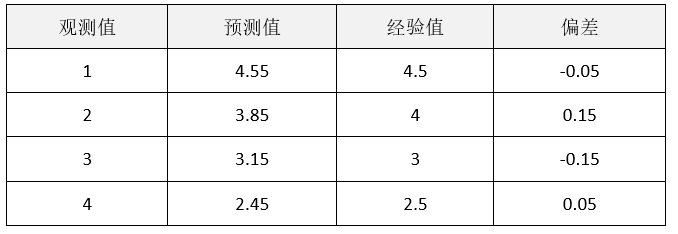2742字，阅读需时10分钟

【摘要：课程通过一个具体案例，借助于EXECL分析工具，讲述线性回归分析必要的步骤和方法，并编写Python程序求出实验数据的拟合函数。】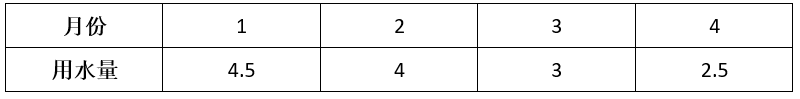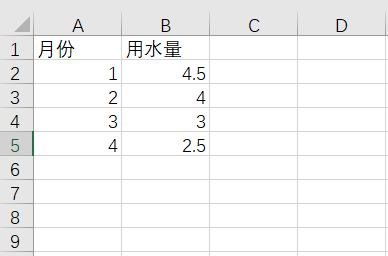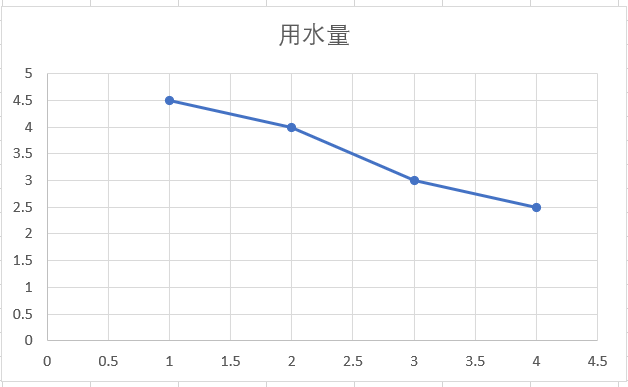`y(i) – f(x(i))  (i=1,2,3,4)`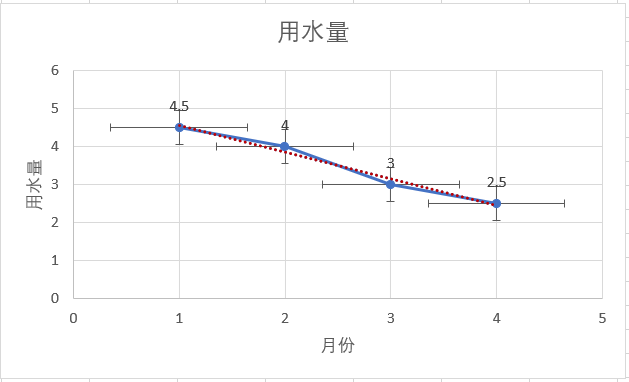`| (y1+y2+y3+y4) -(f(x1)+f(x2)+f(x3)+f(x4)) | = β`

`(y1^2+y2^2+y3^2+y4^2) - (f(x1)^2+f(x2)^2+f(x3)^2+f(x4)^2)  = β``(4.5-(a+b))^2 + (4-(2a+b))^2 + (3-(3a+b))^2 + (2.5-(4a+b))^2 = M`

在上面的公式中，我们希望使所有偏差的平方和最小，如何求最小值M呢？可以通过微积分的方法得到，把偏差的平方和看作函数，它有a和b两个变量，求这个函数的最小值。

```60.0*a + 20.0*b - 63.0 = 0
20.0*a + 8.0*b - 28.0 = 0```

（案例代码见unit1\ case02.py）

```from sympy import diff
from sympy import symbols
# 定义计算偏导的函数
def func(a,b):
return (4.5-(a+b)) ** 2 + \
(4-(2*a+b)) ** 2 + \
(3-(3*a+b)) ** 2 + \
(2.5-(4*a+b)) ** 2
a = symbols("a")
b = symbols("b")
# 计算变量a的偏导函数
print(diff(func(a,b),a))
# 计算变量b的偏导函数
print(diff(func(a,b),b))```

NumPy是Python中科学计算的基础软件包，它提供了众多数学运算工具，这些数学运算工具包括：线性代数中的矩阵和向量运算、傅里叶变换、多维数组运算、数据统计运算以及丰富的数学函数库。

（案例代码见unit1\ case03.py）

```# 导入numpy库
import numpy as np
# 程序入口
if __name__ == '__main__':

# 建立2X2矩阵
ta = np.array([[60.0,20.0],[20.0,8.0]])
# 建立2维向量
tb = np.array([63.0,28.0])
# 解线性方程组
x = np.linalg.solve(ta,tb)
# 输出x和y的值
print("a = %.2f  b = %.2f" % (x,x))

a = -0.70 , b = 5.25
f(x)函数为：
f(x) = -0.7x + 5.25```